# Solve for Y(s), the Laplace transform of the solution y(t) to the initial value problem below... related homework questions

• #### It’s review question, I need this as soon as possible. Thank you 3) For thè diferential equation: (a) The point zo =-1 is an ordinary point. Compute the recursion formula for the coefficients of...It’s review question, I need this as soon as possible. Thank you 3) For thè diferential equation: (a) The point zo =-1 is an ordinary point. Compute the recursion formula for the coefficients of the power series solution centered at zo- -1 and use it to compute the first three nonzero terms of the power series when -1)-s and v(-1)-0....

• #### Dont copié formé thé book oh ya dont copié formé thé book cause you Oiil inde up being triste soi remembré not toi copié frome thé book oh ya

Dont copié formé thé book oh ya dont copié formé thé book cause you Oiil inde up being triste soi remembré not toi copié frome thé book oh ya!translation in english please!

• #### DSuppose \$39oo is deposited in a savings account that increases exponentially.Detamine thě APv if the acount...DSuppose \$39oo is deposited in a savings account that increases exponentially.Detamine thě APv if the acount increases to \$t020 in 4 years. Ass ume tne interest Vale remains Constant and no additional deposits or Withdrawals are made. (a.) Let pbe the APY. Note tnat if tme inital balaqe is yo, ne year later tne balane is %more. P- 3 (Tpe...

• #### Solve initial value problem using Laplace transform Problem 4 Solve the initial value problems given below...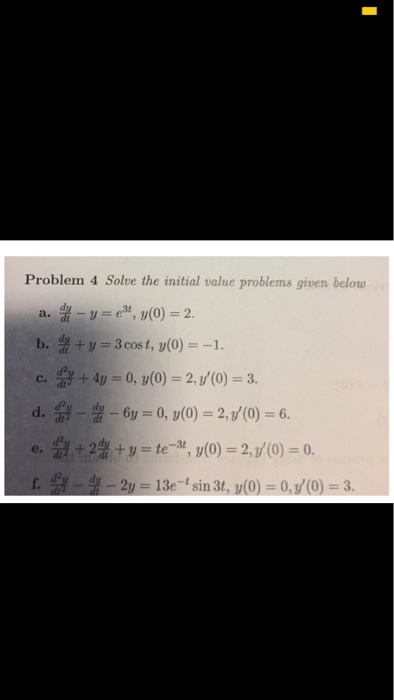Solve initial value problem using Laplace transform Problem 4 Solve the initial value problems given below --ез, y(0) 2. a. b. f ty 3 cos t, y(0)-

• #### 4 points) In this exercise we will use the Laplace transform to solve the following initial value problem: y"-2y, + 17y-17, y(0)-0, y'(0)-1 (1) First, using Y for the Laplace transform of y(t...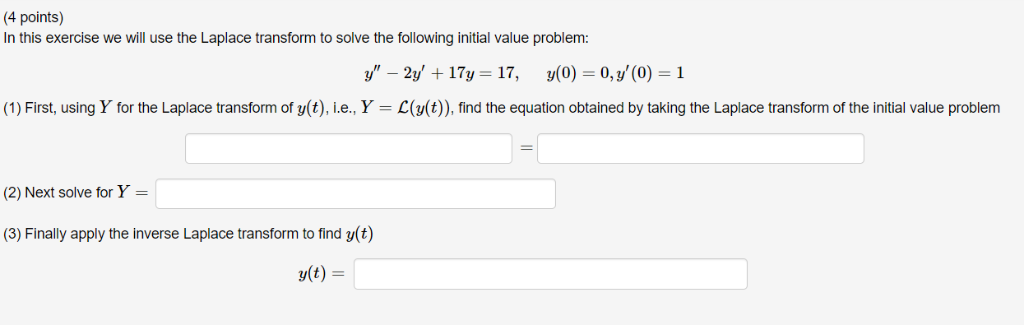4 points) In this exercise we will use the Laplace transform to solve the following initial value problem: y"-2y, + 17y-17, y(0)-0, y'(0)-1 (1) First, using Y for the Laplace transform of y(t), L.e., Y ()), find the equation obtained by taking the Laplace transform of the initial value problem (2) Next solve for Y- (3) Finally apply the inverse...

• #### 7. Using Fourier transform find solution of the following initial value problem: dr2' 46 7. Using Fourier transform find solution of the following initial value problem: dr2' 46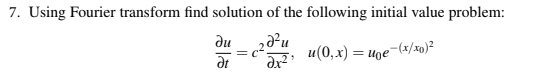7. Using Fourier transform find solution of the following initial value problem: dr2' 46 7. Using Fourier transform find solution of the following initial value problem: dr2' 46

• #### Problem 3. Consider the initial value problem w y sin() 0 Convert the system into a single 3rd order equation and solve resulting initial value problem via Laplace transform method. Express your answ...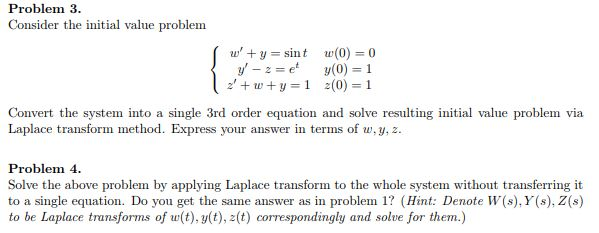Problem 3. Consider the initial value problem w y sin() 0 Convert the system into a single 3rd order equation and solve resulting initial value problem via Laplace transform method. Express your answer in terms of w,y, z. Problem 4 Solve the above problem by applying Laplace transform to the whole system without transferring it to a single equation. Do...

• #### Question (2): Laplace Transforms a) Find the Laplace Transform of the following using the Laplace Transform...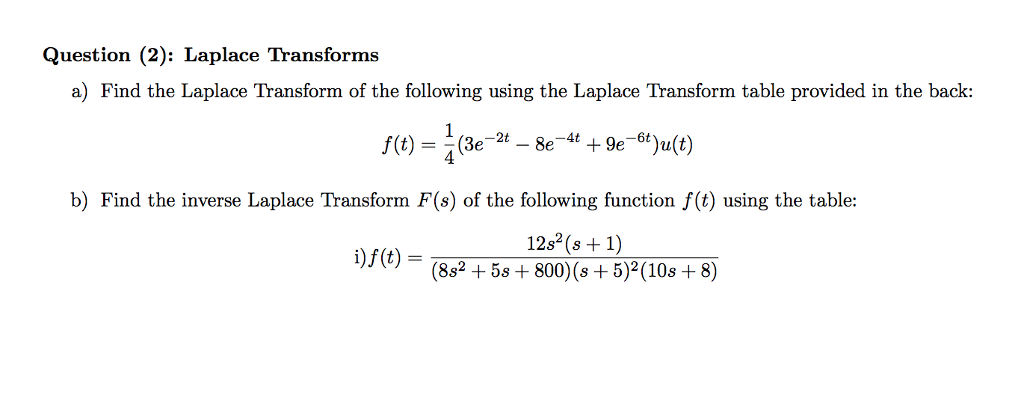Question (2): Laplace Transforms a) Find the Laplace Transform of the following using the Laplace Transform table provided in the back: b) Find the inverse Laplace Transform F(s) of the following function f(t) using the table: 12s (s +1) 1)J (t)-(882 + 5s + 800)(s + 5)2(10s + 8)

• #### Use the Laplace transform to solve the given initial-value problem. Use the table of Laplace transforms...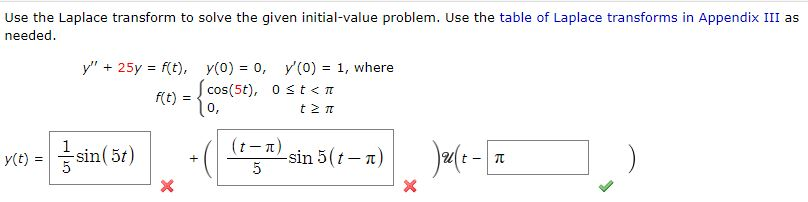Use the Laplace transform to solve the given initial-value problem. Use the table of Laplace transforms in Appendix III as needed. y" + 25y = f(t), y(0) = 0, y (O) = 1, where RE) = {cos(5€), Ostan (Σπ rce) = f sin(51) + (t-1) -sin 5(t-T) 5 Jault- TE ) X

• #### (14) < 4 > Solve by using Laplace transform: y"+9y-30e'; y(0)-0, y' (0) 0 (14) Solve by using Laplace transform: y"+9y-30e'; y(0)-0, y' (0) 0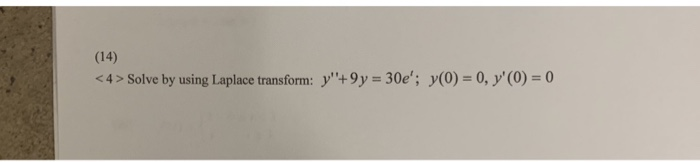(14) < 4 > Solve by using Laplace transform: y"+9y-30e'; y(0)-0, y' (0) 0 (14) Solve by using Laplace transform: y"+9y-30e'; y(0)-0, y' (0) 0

• #### 2. Using Laplace transform, solve the system of differential equations d.x: dy dt where x(0)1 2. Using Laplace transform, solve the system of differential equations d.x: dy dt where x(0)1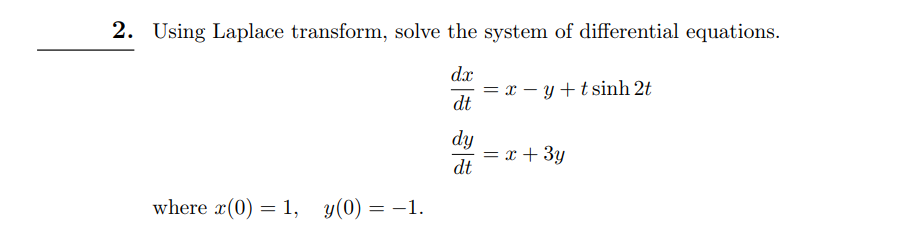2. Using Laplace transform, solve the system of differential equations d.x: dy dt where x(0)1 2. Using Laplace transform, solve the system of differential equations d.x: dy dt where x(0)1

• #### Solve for Y(s), the Laplace transform of the solution y(t) to the initial value problem below...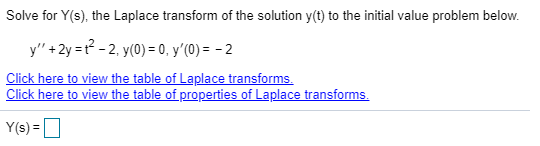Solve for Y(s), the Laplace transform of the solution y(t) to the initial value problem below y+2yt22, y(0) = 0, y'(0) = - 2 Click here to view the table of Laplace transforms Click here to view the table of properties of Laplace transforms Y(s)= Solve for Y(s), the Laplace transform of the solution y(t) to the initial value problem...

• #### Solve for Y(s), the Laplace transform of the solution y(t) to the initial value problem below....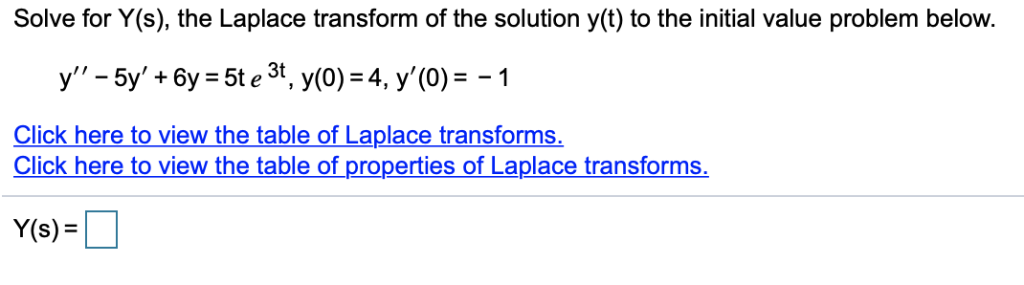Solve for Y(s), the Laplace transform of the solution y(t) to the initial value problem below. Click here to view the table of Laplace transforms. Click here to view the table of properties of Laplace transforms Y(s)

• #### Solve for y(s), the Laplace transform of the solution y(t) to the initial value problem below....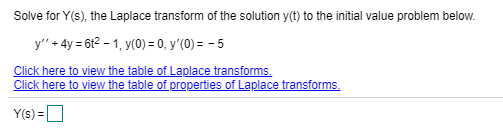Solve for y(s), the Laplace transform of the solution y(t) to the initial value problem below. y'' + 4y = 612 - 1, y(0) = 0, y'(0) = -5 Click here to view the table of Laplace transforms. Click here to view the table of properties of Laplace transforms. Y()=

• #### Solve for Y(s), the Laplace transform of the solution y(t) to the initial value problem below....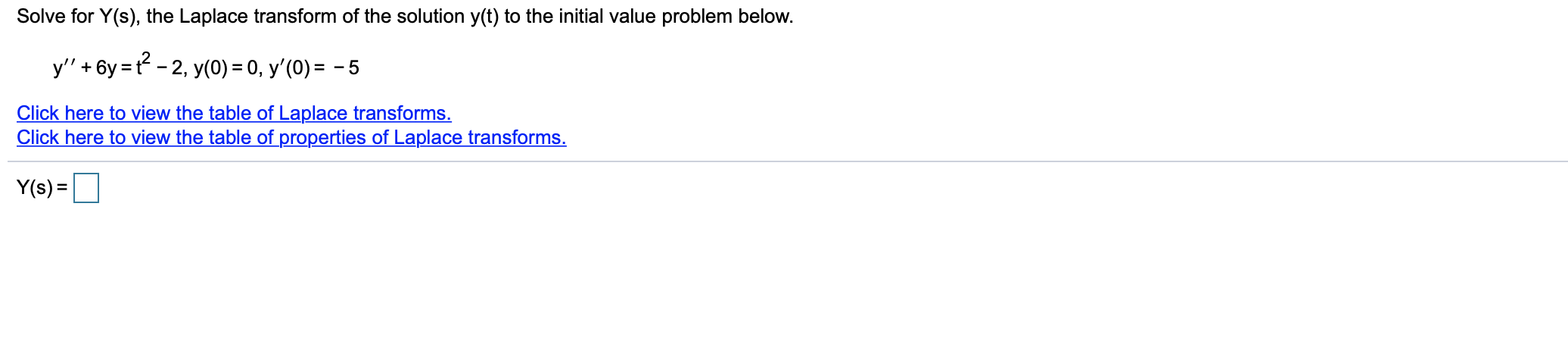Solve for Y(s), the Laplace transform of the solution y(t) to the initial value problem below. y'' +6y=t? - 2, y(0) = 0, y'(0) = -5 Click here to view the table of Laplace transforms. Click here to view the table of properties of Laplace transforms. Y(s)=

• #### Solve for Y(s), the Laplace transform of the solution y(t) to the initial value problem below....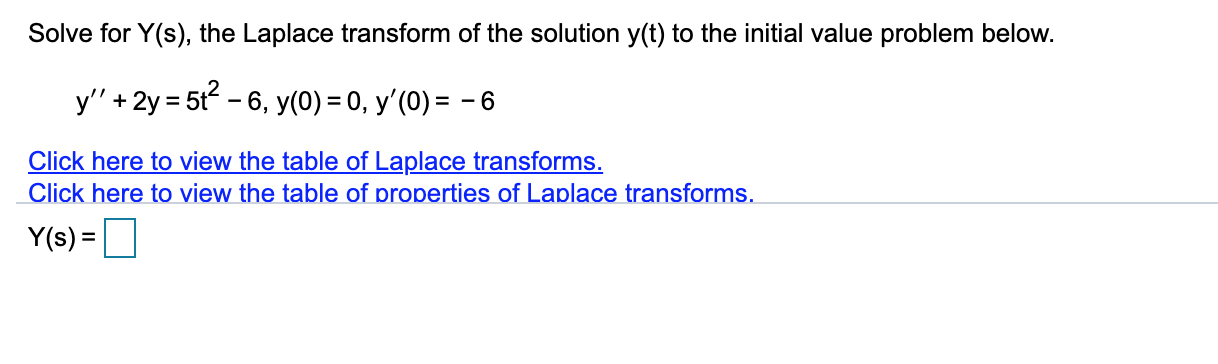Solve for Y(s), the Laplace transform of the solution y(t) to the initial value problem below. y'' + 2y = 562 - 6, y(0) = 0, y'(O) = - 6 Click here to view the table of Laplace transforms. Click here to view the table of properties of Laplace transforms. Y(s) = 1

• #### Solve for Y(s), the Laplace transform of the solution y(t) to the initial value problem below....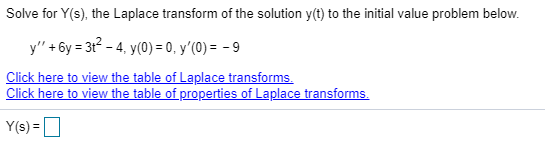Solve for Y(s), the Laplace transform of the solution y(t) to the initial value problem below. y"' + 6y = 3+2 - 4, y(0) = 0, y'(0) = - 9 Click here to view the table of Laplace transforms. Click here to view the table of properties of Laplace transforms. Y(s) =

• #### Solve for Y(s), the Laplace transform of the solution yct) to the initial value problem below....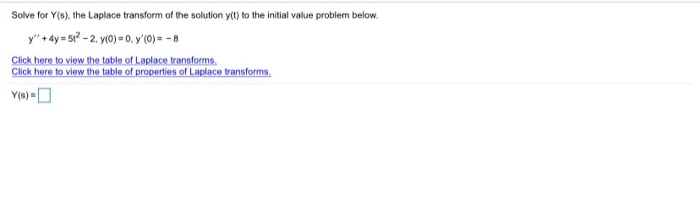Solve for Y(s), the Laplace transform of the solution yct) to the initial value problem below. y" + 4y = 512 - 2. y(0)=0, 7(0) = -8 Click here to view the table of Laplace transforms Click here to view the table of properties of Laplace transforms.

• #### Solve for Y(s), the Laplace transform of the solution y(t) to the initial value problem below....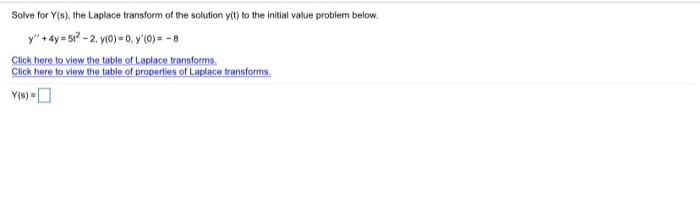Solve for Y(s), the Laplace transform of the solution y(t) to the initial value problem below. y" + 4y = 512 - 2. y(0)=0, 7(0) = -8 Click here to view the table of Laplace transforms Click here to view the table of properties of Laplace transforms. Solve for Y(s), the Laplace transform of the solution y(t) to the initial...

• #### Solve for Y(s), the Laplace transform of the solution y(t) to the initial value problem below....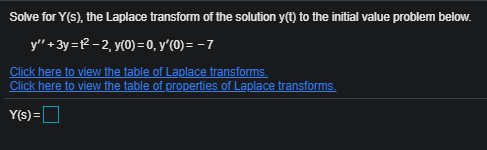Solve for Y(s), the Laplace transform of the solution y(t) to the initial value problem below. y" + 3y = {2-2, y(0) = 0, y'(0) = -7 Click here to view the table of Laplace transforms. Click here to view the table of properties of Laplace transforms. Y(s)=

Free Homework App